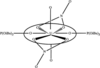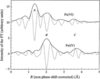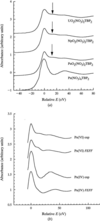## research papersJOURNAL OFSYNCHROTRONRADIATION
ISSN: 1600-5775
Volume 6| Part 2| March 1999| Pages 101-104

## Actinide coordination sphere in various U, Np and Pu nitrato coordination complexes

aCEA, DCC/DRRV/SEMP Laboratoire de Chimie Théorique et Structurale, ValRho, BP 171, 30207 Bagnols sur Cèze, France, bMaterials Science and Technology Division, Los Alamos National Laboratory, Los Alamos, NM 87545, USA, cIPN, Bâtiment 100, Centre Universitaire Paris Sud, 91407 Orsay CEDEX, France, and dCEA, DCC Saclay, 91191 Gif sur Yvette, France
*Correspondence e-mail: denauwer@amandine.cea.fr

(Received 7 October 1998; accepted 5 January 1999)

Waste management of nuclear fuel represents one of the major environmental concerns of the decade. To recycle fissile valuable materials, intimate knowledge of complexation mechanisms involved in the solvent extraction processes is indispensable. Evolution of the actinide coordination sphere of AnO2(NO3)2TBP-type complexes (An = U, Np, Pu; TBP = tributylphosphate) with the actinide valence state have been probed by XAS at the metal LIII edge. Dramatic changes in the actinide coordination sphere appeared when the An(VI) metal is reduced to An(IV). However, no significant evolution in the actinide environment has been noticed across the series UO22+, NpO22+ and PuO22+.

### 1. Introduction

Solvent extraction is the preferred extracting method in the field of nuclear fuel reprocessing. In order to fine-tune the design of selective extractants for better actinide/less-hazardous-waste separation, macroscopic extraction parameters (as kinetics, thermodynamics) must be apprehended. This task might be achieved by a better understanding of the molecular interactions between the chelating extracting ligand and the metal salt to be extracted in the solvent phase. Thus, molecular structural parameters have to be determined in the solvent phase. XAS is a particularly useful probe for both structural and electronic characterization for non-isotropic systems. In this work we present measurements at the LIII edges of actinide ions U, Np and Pu in a series of various liquid-phase coordination complexes involving organophosphate ligands. These ligands are used in liquid extraction processes such as the so-called PUREX process involving tributylphosphate (TBP) for U and Pu recovery (Schulz et al., 1990).

### 2. Experimental

#### 2.1. Sample preparation

Pure TBP (SERLABO) was washed twice (in order to eliminate acidic degradation products) with 0.3 M aqueous Na2CO3 solution and then centrifuged. All complexes were obtained by liquid–liquid extraction with this prepared TBP solution.

The U(VI) complex was prepared according to Den Auwer et al. (1997).

The Np(IV) complex was prepared by reduction of 1.5 ml of 0.02 M Np(V) in HNO3 by zinc amalgam into Np(III). After addition of 1.5 ml of hydrazine (0.1 M) in HNO3 (2.6 M), Np(III) was left to oxidize in air into Np(IV). The aqueous phase was then mixed with 3 ml of the above TBP solution.

The Np(VI) complex was prepared by oxidation of 4 ml of 0.026 M Np(IV) solution in 5 M HNO3 by AgO (black colouration of the solution). The aqueous phase was then mixed with 4 ml of the above TBP solution.

The Pu(IV) complex was prepared by mixing 5 ml of 0.034 M Pu(IV) solution in 2 M HNO3 with 5 ml of the above TBP solution.

The Pu(VI) complex was prepared by oxidation of 4 ml of 0.042 M Pu(IV) solution in 5 M HNO3 by AgO (black colouration of the solution). The aqueous phase was then mixed with 4 ml of the above TBP solution.

In all cases, electronic spectra showed evidence of the expected oxidation states for the U, Np and Pu complexes.

#### 2.2. Data acquisition

Data were acquired on both the D44 beamline of the DCI (1.85 GeV, 300 mA) ring (LURE, France) and the 4–2 beamline of the SPEAR (3 GeV, 100 mA) ring (SSRL, USA). D44 beamline: double-Si(311) fixed-exit monochromator; energy resolution = 6.5 eV at 17000 eV; energy calibration at the Yb LIII edge (17052 eV). 4–2 beamline: double-Si(220) fixed-exit monochromator; energy resolution = 2.0 eV at 17000 eV; energy calibration at the Zr LIII edge (18014 eV).

#### 2.3. Data extraction

Data were extracted using the EXAFS-POWER code (Michalowicz, 1991). A linear function was used for pre-edge extrapolation. Atomic absorption was modelled using a polynomial function of the sixth power. Normalization was achieved using the Heitler–Heisenberger method. The Fourier transform [k3χ(k)] was calculated with the Kaiser window (τ = 2.5) between 2.5 and 13.7 Å−1. Back-Fourier transforms were performed in the ranges 0.9–2.6 Å for AnO2(NO3)2TBP2 complexes and 1.5–3.0 Å for An(NO3)4TBP2 complexes.

Adjustments were carried out using the FEFFIT code (Newville, 1995) using phases, amplitudes and the electron mean free path calculated by code FEFF7.02 on the basis of UO2(NO3)2(TiBP)2 solid-state cluster calculations. Details of the electronic parameters used for the calculations are given elsewhere (Den Auwer et al., 1998).

Amplitude parameters N (number of neighbours), S02 (multi-electronic losses) and e0 (energy threshold) were not fitted; R (An—X distance, X = O, N) and σ (Debye–Waller factor) were adjusted. N was fixed according to the molecular models used and described in Table 1. S02 and e0 were fixed for all complexes at the values determined by the fit in identical conditions of model solid-state compound UO2(NO3)2(TiBP)2 (S02 = 0.851 and e0 = 1.99 eV). Debye–Waller factors were kept constant between all species of related adducts [i.e. AnO2(NO3)2(TBP)2 on one hand and An(NO3)4(TBP)2 on the other]; this approximation allows a better comparison of the bond distances within the series An = U, Np and Pu.

 Table 1Structural models used in the fitting procedure of AnO2(NO3)2(TBP)2 and An(NO3)4(TBP)2 complexes
Complex type Neighbours Range ofexpecteddistances
AnO2(NO3)2(TBP)2 Two O(axial) 1.8 Å
Four O(nitrates) 2.5 Å
Two O(phosphate) 2.4 Å
Two N(nitrates) 3.0 Å
An(NO3)4(TBP)2 model 1 Two O(phosphate) 2.4 Å
Four O(monodendate NO3) <2.5 Å
Four N(nitrates) 3.7 Å
An(NO3)4(TBP)2 model 2 Two O(phosphate) 2.4 Å
Eight O(bidendate NO3) 2.5 Å
Four N(nitrates) 3.0 Å
†Den Auwer et al. (1998).

### 3. Results and discussion

In this paper, two series of similar adducts are considered. The first involves AnO22+(VI) (An = U, Np, Pu) nitrate coordination with TBP ligand in TBP and the second deals with the corresponding adducts in which the actinide formal oxidation state is IV.

We first consider here the series of U, Np and Pu complexes with the general formula AnO2(NO3)2(TBP)2 in TBP solution. The coordination sphere of the solid-state reference complex UO2(NO3)2(TiBP)2 (where TiBP = triisobutylphosphate) can be schematized as in Fig. 1(Burns & Brown, 1985) and compares with the An(VI) species polyhedron. Simulation of the EXAFS spectrum of this complex for electronic parameter calculations can be satisfactorily achieved using the FEFF code (Hudson et al., 1995; Den Auwer et al., 1998). In addition, paths analysis of the spectrum reveals that only five major single-scattering paths can reproduce the filtered EXAFS data (between 0.9 and 2.6 Å). Recent calculation studies have shown a slight decrease in covalency from U to Pu adducts (Hirata et al., 1998). However, An—O(phosphate) distances are very unlikely to be affected by such a small overlap density variation. Table 2indeed shows that no significant bond-length evolution occurs across the series.

 Table 2Best-fit parameters [R (Å) and σ (Å)] of the EXAFS spectra of AnO2(NO3)2(TBP)2 (An = U, Np, Pu) solution complexes at room temperature
Neighbours U Np Pu
An—O, R = 1.77 ± 0.01, R = 1.75 ± 0.01, R = 1.75 ± 0.01,
N = 2 σ = 0.04 ± 0.02 σ = 0.04 σ = 0.04
An—O(P), R = 2.41 ± 0.01, R = 2.38 ± 0.02, R = 2.40 ± 0.02,
N = 2 σ = 0.06 ± 0.04 σ = 0.06 σ = 0.06
An—O(N), R = 2.54 ± 0.01, R = 2.51 ± 0.01, R = 2.50 ± 0.01,
N = 2 σ = 0.07 ± 0.04 σ = 0.07 σ = 0.07
An—O′(N), R = 2.54 ± 0.01, R = 2.51 ± 0.01, R = 2.50 ± 0.01,
N = 2 σ = 0.07 ± 0.04 σ = 0.07 σ = 0.07
An . . . N, R = 2.98 ± 0.03, R = 2.90 ± 0.03, R = 2.91 ± 0.03,
N = 2 σ = 0.06 ± 0.05 σ = 0.06 σ = 0.06
R-factor 0.02 0.03 0.03Figure 1 Schematic representation of the UO2(NO3)2(TBP)2 complex.

EXAFS spectra of the Pu and Np adducts with formal oxidation states equal to IV have been recorded [the U(IV) nitrate complex is not stable]. In Fig. 2, as an example, EXAFS spectra of the Pu(VI) and Pu(IV) complexes show the disappearance of the axial O atoms upon reduction of Pu(VI) to Pu(IV). Contribution A, related to the 1.75 Å Pu—O axial bond, is absent in the Pu(IV) spectrum. In both complexes, contribution B accounts for the Pu—O(nitrate) and Pu—O(phosphate) bonds. As identified in previous studies, contribution C is attributed to backscattering of the two P atoms, showing that the Pu—O(phosphate) bond length is not dramatically changed upon reduction of the Pu oxidation state. Two structural models may be considered (Table 1) for the Np(IV) and Pu(IV) species: the first one (model 1) consists of two oxygen-bridged TBP ligands and four monodendate nitrates; model 2 consists of the two oxygen-bridged TBP ligands and four bidendate nitrates. The fitting procedure according to both models for the Pu and Np adducts leads unambiguously to discarding model 1. Table 3compares the best-fit parameters obtained for both Pu and Np adducts according to model 2. Comparable bond distances are found in both Np and Pu cases, confirming the similarity of these complexes as in the case of AnO2(NO3)2(TBP)2 complexes (Table 2). Moving from AnO2(NO3)2(TBP)2 complexes (An = Np, Pu) to An(NO3)4(TBP)2 complexes, the An—O bond distances seem to be slightly decreasing (even if the difference remains within the error bars). This would be in agreement with an increase in the electrostatic charge moving from the AnO22+ species to the An4+ ions and/or a decrease in the steric effect of the axial O atoms around the An atom, resulting in an increase in the An—O interaction.

 Table 3Best-fit parameters [R (Å) and σ (Å)] of the EXAFS spectra of both Pu(VI) and Pu(IV) TBP solution species at room temperature
Neighbours Np (IV) Pu (IV)
An—O(P), R = 2.35 ± 0.02, R = 2.38 ± 0.01,
N = 2 σ = 0.07 ± 0.04 σ = 0.07
An—O(N), R = 2.50 ± 0.01, R = 2.48 ± 0.01,
N = 8 σ = 0.08 ± 0.03 σ = 0.08
An . . . N, R = 2.96 ± 0.02, R = 2.95 ± 0.01,
N = 4 σ = 0.07 ± 0.04 σ = 0.07
R-factor 0.02 0.02Figure 2 Fourier transform (FT) (modulus and imaginary part) of the EXAFS spectra of solution complexes PuO2(NO3)2(TBP)2 and Pu(NO3)4(TBP)2.

In Fig. 3(a), XANES spectra at the An LIII edge of the three actinide complexes show the characteristic resonance feature (indicated by an arrow in the figure) that accounts for multiple scattering within the actinyl rod. This resonance has been shown to be attributed to triple and quadruple multiple-scattering paths along the rod (Den Auwer et al., 1998; Ankudinov & Conradson, 1998). In the case of UO2(NO3)2(TBP)2, the sudden decrease of the white-line intensity is attributed to the decrease of the D44 beamline resolution compared with the 4–2 beamline where NpO2(NO3)2(TBP)2 and PuO2(NO3)2(TBP)2 were recorded. Upon reduction of the actinide oxidation state from VI to IV (here shown in the case of Pu adducts), the resonance vanishes (Fig. 3b), as can be simulated by the FEFF code using the simple first-approximation model 2 complex of Pu(IV).Figure 3 (a) LIII edges of the AnO2(NO3)2(TBP)2 solution complexes (An = U, Np, Pu) and Pu(NO3)4(TBP)2. (b) Experimental (exp) and calculated (FEFF) LIII edges of PuO2(NO3)2(TBP)2 and Pu(NO3)4(TBP)2 solution species. Edge energies are renormalized versus the white-line maximum of the An(VI) species.

### 4. Conclusions

In this work, An(VI)O2(NO3)2(TBP)2 solution complexes are shown to be isostructural across the U, Np and Pu series. No significant An—O bond-length change has been observed at the An LIII edge. Upon reduction of the An oxidation state (An = Pu, Np) from VI to IV, evidence of the disappearance of the axial O atoms is given by both EXAFS and XANES investigations, while adjustment of the structural EXAFS parameters account for the Pu—O(nitrate) and Pu—O(phosphate) coordination sphere evolution.

### Footnotes

LURE (Laboratoire d'Utilisation du Rayonnement Electromagnétique, Université Paris Sud, France) associate member.

### Acknowledgements

We would like to thank Dr Ph. Moisy and J. P. Dognon for fruitful discussions and advice on An synthesis and model cluster construction. We would like to acknowledge the COGEMA company for funding.

### ReferencesJOURNAL OFSYNCHROTRONRADIATION